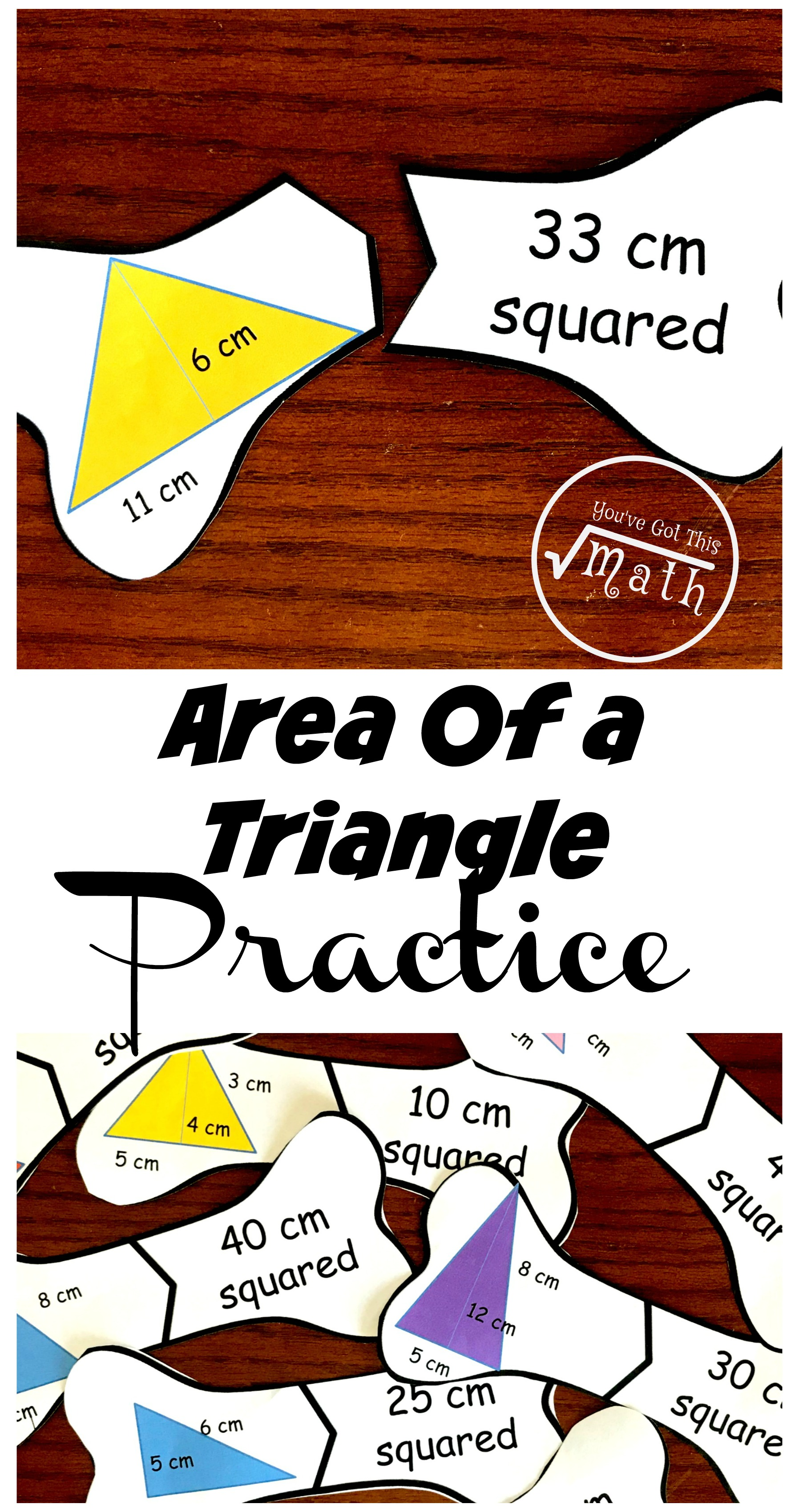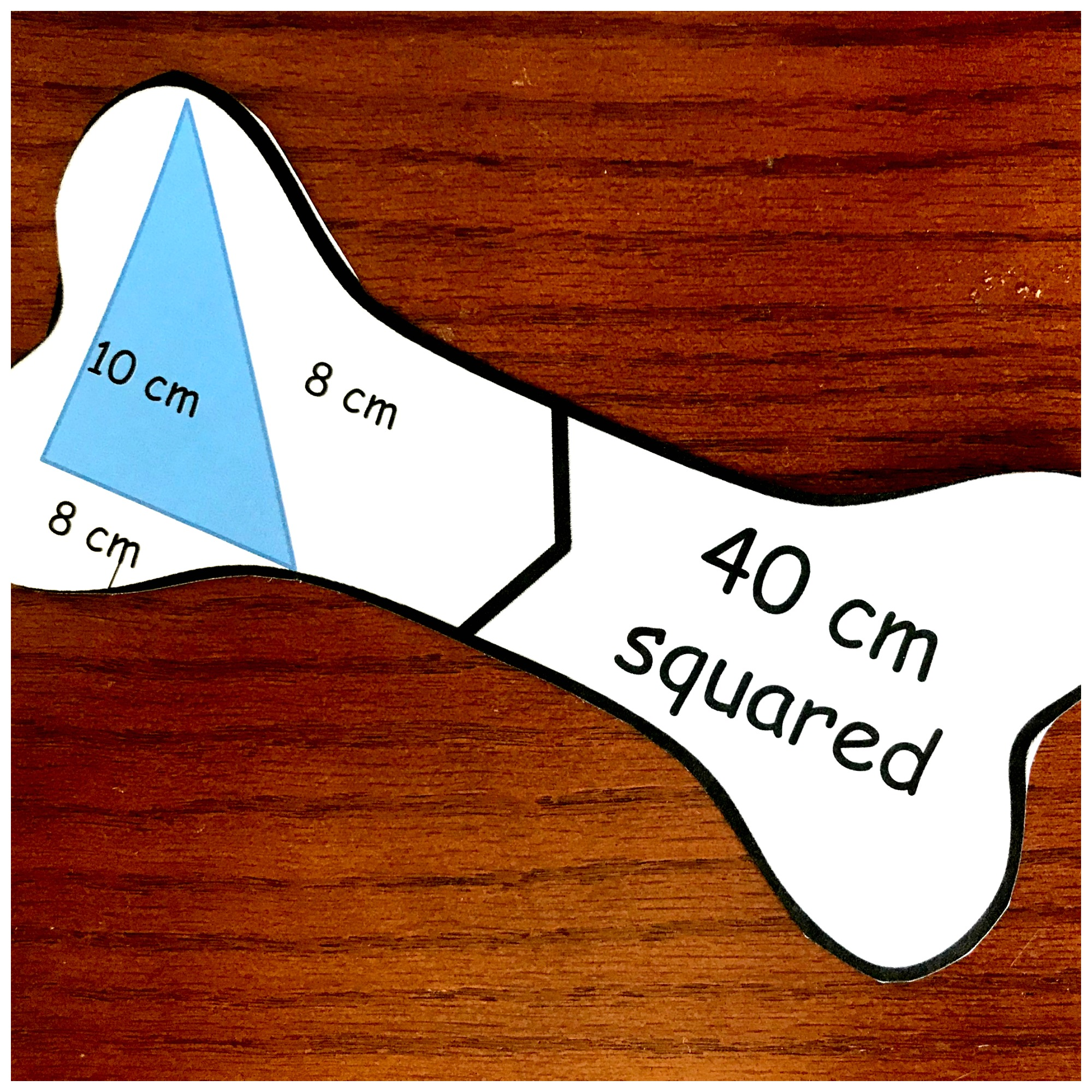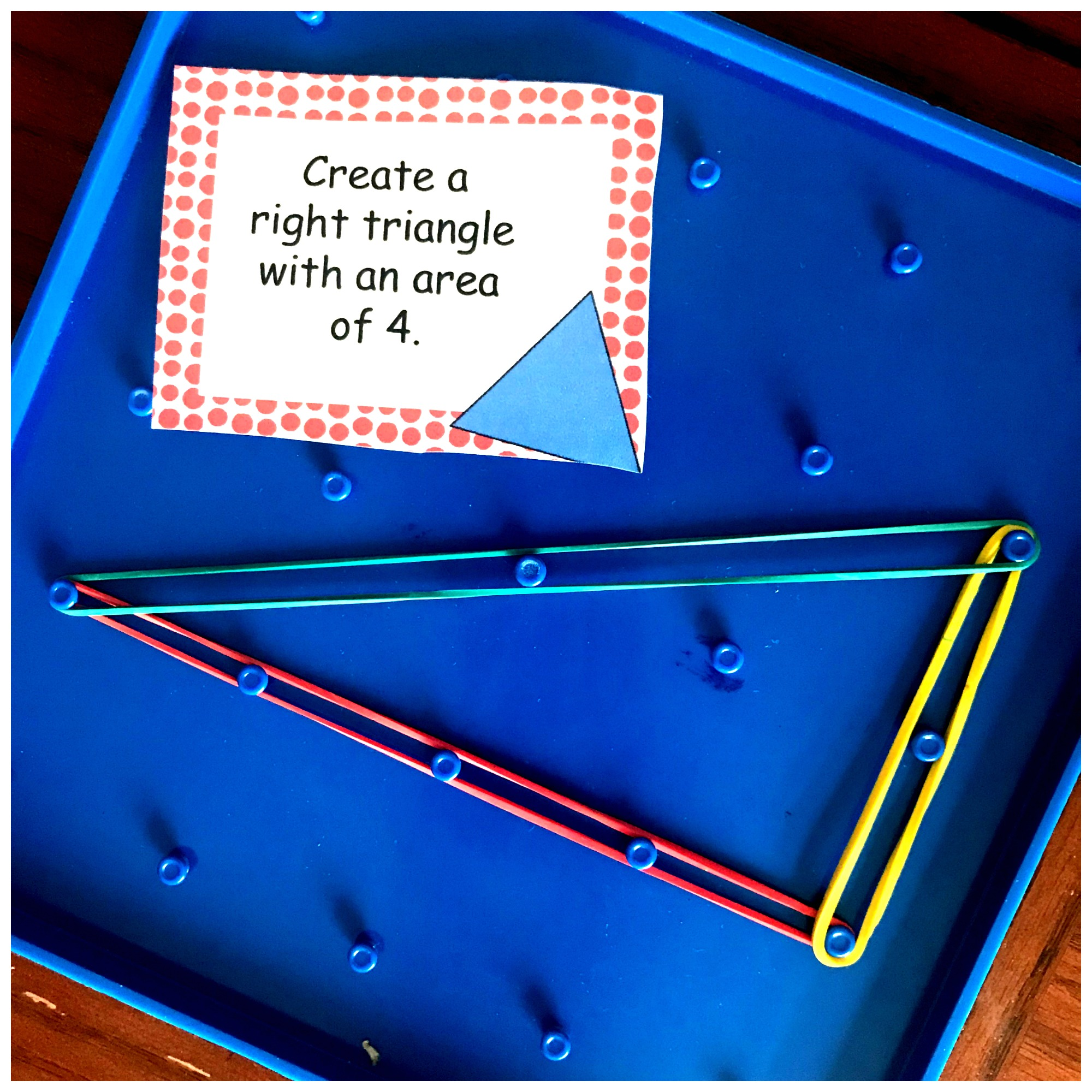Home » Math » 10 Helpful Puzzles to Provide Area of Triangles Practice

# 10 Helpful Puzzles to Provide Area of Triangles Practice

These puzzles provide some fun area of a triangle problems. They are a great alternative to worksheets and allow children to get in extra practice.Over the past few days, my little guys have memorized the area of a triangle, did an interactive notebook to help them understand the formula, and built triangles with specific areas. And today it is time for a little area of a triangle practice.

And how will we practice? By putting together bone puzzles…of course. So grab some scissors and join us in a little fun.

## Prep-Work

1. Begin by printing off the puzzles on card stock paper.
2. Next, cut them out.
3. Provide dry erase markers and a whiteboard so the students can work out these area of a triangle problems.Charles Leonard Dry Erase Lapboard Class Pack, Includes 12 each of Whiteboards, 2 Inch Felt Erasers and Black Dry Erase Markers (35036)## Directions for Area of a Triangle Problems

There are two types of area problems provided.

The first ones focus on right triangles. These are a little easier because the children can see the height. When figuring the area of the triangle you must figure out the height, and the height must be a straight line. Since right triangles have two straight lines it is easy to see the base and the height.In this example, you can see that the base is 8 and the height is 10. When we put those numbers into a formula it looks like this.

1/2 x 8 x 10

1/2 x 80

40

If your children are struggling with multiplying by 1/2, this post may work)

The other set allows children to experience other triangles.In this example, you can see that the base is 11 and the height is 6. It is important for your children to understand where to find the height of a triangle, and that it may not always be its side.

Once again we plug in the numbers to our formula 1/2 b x h.

1/2 x 11 x 6

1/2 x 66

33

I hope your children enjoy practicing area of triangle problems with these puzzles.

You may also like:

Interactive NotebookArea of a Triangle STEM Cards